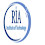# TestNG Priority and Sequencing

Hi everyone, in this post we will see how to priorities Test cases in TestNG and how to sequence it as per our requirement. If you create a test class and add multiple test cases and run it then each time test case execution sequence is randomly selected .
With TestNG annotations @Test and parameter "priority" we can define sequence and priority for executing test cases. Let us consider the below example and if you run the test class given below multiple times you can see that each time execution sequence/flow is different hence to define a fixed execution flow we user TestNG annotation priority.

### Video Tutorial -

```package test;
import org.testng.annotations.Test;

public class TesNGSequencing {

@Test

public void One() {

System.out.println("Test Case number One");

}

@Test

public void Two() {

System.out.println("Test Case number Two");

}

@Test

public void Three() {

System.out.println("Test Case number Three");

}

@Test

public void Four() {

System.out.println("Test Case number Four");

}

}
```

Given below is the example for using priority annotations. When you run the below test class then each time execution flow is constant and starts with test case having 0 priority.

```package test;
import org.testng.annotations.Test;

public class TesNGSequencing {

@Test(priority=0)

public void One() {

System.out.println("Test Case number One");

}

@Test(priority=1)

public void Two() {

System.out.println("Test Case number Two");

}

@Test(priority=2)

public void Three() {

System.out.println("Test Case number Three");

}

@Test(priority=3)

public void Four() {

System.out.println("Test Case number Four");

}

}
```

### Skipping a Test -

If you come across a situation where there is requirement to skip some tests then in this case you can do it using @Test annotations and enabled parameter.

```package test;
import org.testng.annotations.Test;

public class TesNGSequencing {

@Test(priority=0, enabled=false)

public void One() {

System.out.println("Test Case number One");

}

@Test(priority=1)

public void Two() {

System.out.println("Test Case number Two");

}

@Test(priority=2)

public void Three() {

System.out.println("Test Case number Three");

}

@Test(priority=3)

public void Four() {

System.out.println("Test Case number Four");

}

}
```

1.2.